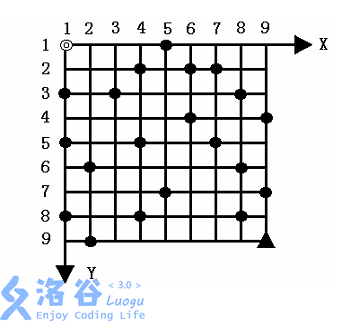# 【网络流24题】汽车加油行驶问题

## 【网络流24题】汽车加油行驶问题N,K,A,B,C 均为正整数， 且满足约束: 2≤N≤100,2≤K≤10 。

// luogu-judger-enable-o2
#include<bits/stdc++.h>
const int N = 110;
int dis[N][N];
int map[N][N];
int n,k,a,b,c;
int v[N][N];
int dx={0,-1,0,1};
int dy={-1,0,1,0};
struct heap {
int x,y,d,v;
bool operator < (const heap m) const{
return v>m.v;
//if(d!=m.d)
//return d<m.d;
}
};
int main (){
scanf("%d %d %d %d %d",&n,&k,&a,&b,&c);
for(int i=1;i<=n;++i){
for(int j=1;j<=n;++j){
scanf("%d",&map[i][j]);
}
}
memset(dis,0x3f,sizeof(dis));
dis[k]=0;
std::priority_queue<heap> q;
q.push((heap){1,1,k,0});
int cnt=0;
while(!q.empty()){
cnt++;
heap x=q.top();q.pop();
if(v[x.x][x.y][x.d])continue;
v[x.x][x.y][x.d]=1;
int cost=a;
if(map[x.x][x.y]==1&&x.d!=k){
if(dis[x.x][x.y][k]<=dis[x.x][x.y][x.d]+cost) continue;
dis[x.x][x.y][k]=dis[x.x][x.y][x.d]+cost;
q.push((heap){x.x,x.y,k,dis[x.x][x.y][k]});
continue;
}
cost+=c;
if(dis[x.x][x.y][k]>dis[x.x][x.y][x.d]+cost){
dis[x.x][x.y][k]=dis[x.x][x.y][x.d]+cost;
q.push((heap){x.x,x.y,k,dis[x.x][x.y][k]});
}
if(x.d<=0) continue;
for(int i=0;i<=3;++i){
int gx=x.x+dx[i];
int gy=x.y+dy[i];
if(gx>n||gy>n||gx<1||gy<1) continue;
int cost=0;
if(i<=1) cost=b;
if(x.d!=0&&(dis[gx][gy][x.d-1]>(dis[x.x][x.y][x.d]+cost))){
dis[gx][gy][x.d-1]=dis[x.x][x.y][x.d]+cost;
q.push((heap){gx,gy,x.d-1,dis[gx][gy][x.d-1]});
}
}

}
int ans=0x3f3f3f3f;
for(int i=0;i<=k;++i){
ans=std::min(ans,dis[n][n][i]);
//printf("%d\n",dis[n][n][i]);
}
// printf("%d\n",dis[k]);
printf("%d",ans);
return 0;
}



### 一条评论

1.wjyyy 2018年7月6日 下午9:11 回复

到油站必须加油啊。。。？

Ɣ回顶部